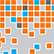# Online Program

Friday, May 31The Cutting Edge in Statistical Machine Learning
Fri, May 31, 1:30 PM - 3:05 PM
Regency Ballroom AB

### A Continuous-Time View of Early Stopping in Least Squares Regression (305009)

Alnur Ali, Carnegie Mellon University
Zico Kolter, Carnegie Mellon University
*Ryan Tibshirani, Carnegie Mellon University

Keywords: gradient flow, ridge regression, early stopping, implicit regularization, random matrix theory

We study the statistical properties of the iterates generated by gradient descent, applied to the fundamental problem of least squares regression. We take a continuous-time view, i.e., consider infinitesimal step sizes in gradient descent, in which case the iterates form a trajectory called gradient flow. In a random matrix theory setup, which allows the number of samples n and features p to diverge in such a way that p/n converges to a positive constant, we derive and analyze an asymptotic risk expression for gradient flow. In particular, we compare the asymptotic risk profile of gradient flow to that of ridge regression. When the feature covariance is spherical, we show that the optimal asymptotic gradient flow risk is between 1 and 1.25 times the optimal asymptotic ridge risk. Further, we derive a calibration between the two risk curves under which the asymptotic gradient flow risk no more than 2.25 times the asymptotic ridge risk, at all points along the path. Lastly, we present numerical experiments that show ridge and gradient flow to be extremely tightly coupled, even more so than the theory predicts.